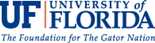# Arduinos: Morse Code

Physical Computing, the Internet of Things, Sensors and Servos

## Goals

Goals

• Learn how to write to the Serial Monitor to affect changes to your prototype
• Learn how to write functions dot(), dash(), and soundLetter()
• Learn how to use tone() to create simple sounds out of piezo
• Learn about Morse code and use it to send light and sound messages

## Arduino Code

/*
Piezo Element Morse Code Sketch
http://www.multiwingspan.co.uk/arduino.php?page=buzzer2
1-piezo
1- LED
1-220 ohm resistor
*/

const int piezoPin = 9;
const int ledPin = 13;
// tone frequency C
const int tonefreq = 523;

// constants for tone and rest durations
const int dotlength = 100;
const int dashlength = dotlength * 3;
// inter-element gap - between each dot or dash of a letter
const int inter = dotlength;
// letter gap is 3 dots - the inter gap is always added - so this is one fewer
const int lgap = dotlength * 2; // inter-letter gap
// word gap is 7 dots - with letter and inter gap already counted, this is -1
const int wgap = dotlength * 4; //inter-word gap

void setup()
{
pinMode(piezoPin, OUTPUT);
pinMode(ledPin, OUTPUT);
Serial.begin(9600);
}

void loop()
{
char thischar;
if (Serial.available())
{
if (thischar>='a' && thischar<='z')
{
// convert to upper case
thischar = thischar -32;
}
// swap for a space if not in A-Z
if(thischar<65 || thischar>90)
{
thischar=' ';
}
soundLetter(thischar);
delay(lgap);
}
}

void dot()
{
// play a dot
tone(piezoPin, tonefreq);
// LED
digitalWrite(ledPin, HIGH);
delay(dotlength);
noTone(piezoPin);
// LED
digitalWrite(ledPin, LOW);
delay(inter);
}

void dash()
{
// play a dash
tone(piezoPin, tonefreq);
// LED
digitalWrite(ledPin, HIGH);
delay(dashlength);
noTone(piezoPin);
// LED
digitalWrite(ledPin, LOW);
delay(inter);
}

void soundLetter(char letter)
{
// letters are in alphabetic order
switch(letter)
{

case 'A':
dot();
dash();
return;
case 'B':
dash();
dot();
dot();
dot();
return;
case 'C':
dash();
dot();
dash();
dot();
return;
case 'D':
dash();
dot();
dot();
return;
case 'E':
dot();
return;
case 'F':
dot();
dot();
dash();
dot();
return;
case 'G':
dash();
dash();
dot();
return;
case 'H':
dot();
dot();
dot();
dot();
return;
case 'I':
dot();
dot();
return;
case 'J':
dot();
dash();
dash();
dash();
return;
case 'K':
dash();
dot();
dash();
return;
case 'L':
dot();
dash();
dot();
dot();
return;
case 'M':
dash();
dash();
return;
case 'N':
dash();
dot();
return;
case 'O':
dash();
dash();
dash();
return;
case 'P':
dot();
dash();
dash();
dot();
return;
case 'Q':
dash();
dash();
dot();
dash();
return;
case 'R':
dot();
dash();
dot();
return;
case 'S':
dot();
dot();
dot();
return;
case 'T':
dash();
return;
case 'U':
dot();
dot();
dash();
return;
case 'V':
dot();
dot();
dot();
dash();
return;
case 'W':
dot();
dash();
dash();
return;
case 'X':
dash();
dot();
dot();
dash();
return;
case 'Y':
dash();
dot();
dash();
dash();
return;
case 'Z':
dash();
dash();
dot();
dot();
return;
case ' ':
delay(wgap);
return;
}
}

## Hardware schematicsIn this set up, imagine that we have two circuits. Both start and end at the Arduino. In the first, we take a wire from digital GND to the breadboard to ground the circuit. We then use the same row to connect one leg of our resistor. The second leg of the resistor should be in a separate row and connect to the short leg of the LED. The long leg of the LED should be in a different row and should have a jumper wire connecting back to digital pin 13, which will control when the light blinks. The second circuit can use the same wire from ground to the breadboard. It should then connect a wire from that grounded row to a separate row. That new row should connect to the black wire of the piezo. The red wire should be in a separate row. There should be a new jumper wire connecting the last row back to digital pin 9 to control the tones beeping. (NOTE: There is more than one correct way to build a circuit. You could separately ground each circuit.)

## Morse Code Chart## ASCII Table## Electronic Components

Input

For input, we will use the Serial Monitor, so no electronic components are required.

Output

For output, we will also be using an analog piezo element and a light-emitting diode (LED). When you send electricity through a piezo, it vibrates at certain frequencies which produces tones. LEDs are unidirectional: the long lead is positive, the short lead is negative.

Resistors

To reduce the amount of current, we will be using one 220 ohm resistor. Resistors reduce current flow and lower voltage. The amount of resistance provided is color coded. You can always use the color chart below to determine resistance. To read more about resistors, check out https://learn.sparkfun.com/tutorials/resistors .